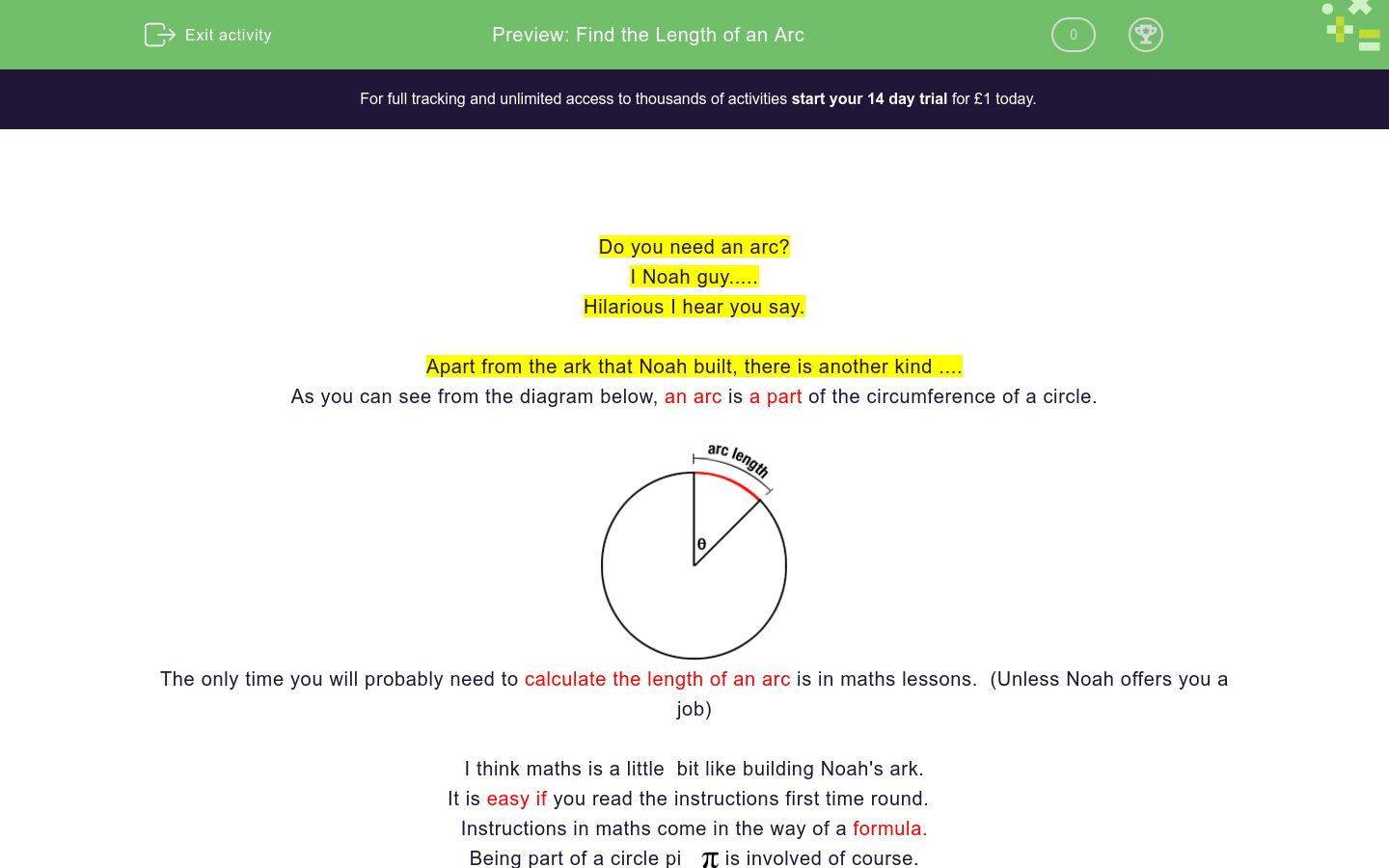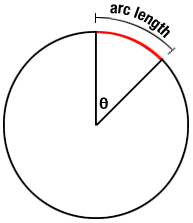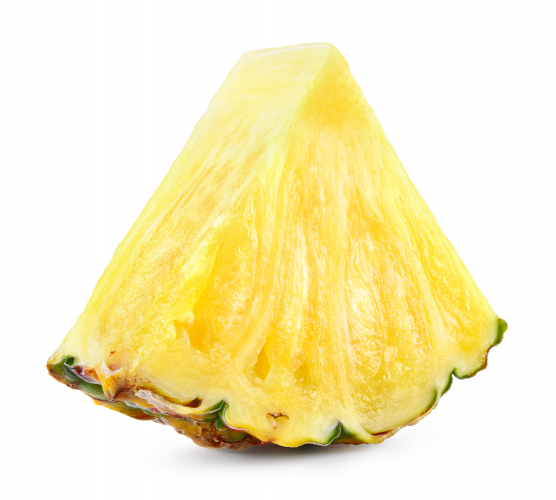# Find the Length of an Arc

In this worksheet, students will learn the formula for finding the length of an arc and will be able to apply it.Key stage:  KS 4

GCSE Subjects:   Maths

GCSE Boards:   AQA, Eduqas, OCR, Pearson Edexcel

Curriculum topic:   Geometry and Measures, Mensuration

Curriculum subtopic:   Mensuration and Calculation, Perimeter Calculations

Difficulty level:### QUESTION 1 of 10

Do you need an arc?

I Noah guy.....

Hilarious I hear you say.

Apart from the ark that Noah built, there is another kind ....

As you can see from the diagram below, an arc is a part of the circumference of a circle.The only time you will probably need to calculate the length of an arc is in maths lessons.  (Unless Noah offers you a job)

I think maths is a little  bit like building Noah's ark.

It is easy if you read the instructions first time round.

Instructions in maths come in the way of a formula.

Being part of a circle piis involved of course.Also if you know how to find the circumference of a circle we have a bit of a gift.

Circumference of a circle is Π x diameter  (πd)

As an arc is part of the circumference it makes sense to use this as part of our calculation.

Length of an arc = angle ÷ 360 x π x diameter

Generally in maths questions your are given the radius of the circle, you could double the radius to get the diameter or quite often the formula applied could be..

Length of an arc = angle ÷ 360 x 2 x π x radius

Remember you can us the π button on your calculator or use the values 3.14 or 3.142Find the length of the red arc.

The angle at the centre is  63°.

6.60 cm

7.20 cm

8.40 cm

5.60 cmWhat is the length of the blue arc?

The radius is 6 cm and the angle at the centre of the circle is 130°.Given that the radius of this circle is 11 cm and the angle at the centre is 96°

Calculate the length of the arc formed by the white lights on this speedometer.

15.29 cm

11.68 cm

17.54 cm

18.43 cnThe radius of this pineapple is 7 cm.

The angle at the centre is 30°

15.29 cm

11.68 cm

17.54 cm

18.43 cnCalculate the arc length of one of the green sections of this dartboard.

The angle at the centre of each section is 18° and the radius is 8.5 cmThe angle at the centre of the yellow arc is 120° The radius is 3.2 cm.Calculate the arc length of the light blue section of this pie chart.

The angle is 78° and the radius of the pie chart is 12 cm.Given that the radius of this circle is 6.5 cm and the angle at the centre is 47° calculate the arc length from one point of the triangle to the other.

 4.62 cm 6.56 cm 5.33 cm 7.21cm arc length isGiven that the angle at the centre is 59° and the radius is 5 cm.

Calculate the arc length.

So Noah did things in two's.  So lets match up the dimensions with the arc lengths.

## Column B

7.94 cm
4.99 cm
4.95 cm
5.03 cm
• Question 1Find the length of the red arc.

The angle at the centre is  63°.

6.60 cm
EDDIE SAYS
Its a bit like Noah putting animals into his ark. You just have to put the numbers into the formula to find the length of your arc. 63÷ 360 x π x 2 x 6 (or 63÷ 360 x π x 12)
• Question 2What is the length of the blue arc?

The radius is 6 cm and the angle at the centre of the circle is 130°.

13.61
EDDIE SAYS
130 ÷360 x π x 2 x 6 = 13.61 cm correct to 2 decimal places Keep practicing the formula it really is the key to unlocking things about the arc.
• Question 3Given that the radius of this circle is 11 cm and the angle at the centre is 96°

Calculate the length of the arc formed by the white lights on this speedometer.

18.43 cn
EDDIE SAYS
I wonder if Noah has a speedometer in his ark. 96 ÷ 360 x π x 2 x 11 = 18.43cm Do you think Noah was speeding?
• Question 4The radius of this pineapple is 7 cm.

The angle at the centre is 30°

EDDIE SAYS
I bet you never considered the maths in a piece of pineapple before did you. So our arc length for this is 30 ÷360 x π x 2 x 7 = 3.67 cm That a bit small for my liking.
• Question 5Calculate the arc length of one of the green sections of this dartboard.

The angle at the centre of each section is 18° and the radius is 8.5 cm

2.67
EDDIE SAYS
18 ÷ 360 x π x 2 x 8.5 = 2.67 cm to (2 d.p) This is great, I can work out an arc length, but never hit the dartboard. I hope you got a bullseye.
• Question 6The angle at the centre of the yellow arc is 120° The radius is 3.2 cm.

EDDIE SAYS
I think Noah got around a bit. I wonder if the ark made it to Spain. Let us find the arc length. 120 ÷ 360 x π x 2 x 3.2 = 6.70 cm
• Question 7Calculate the arc length of the light blue section of this pie chart.

The angle is 78° and the radius of the pie chart is 12 cm.

16.34
EDDIE SAYS
I hope you are getting used to applying the formula. it is a bit like being an arc angel in our maths work. 78 ÷ 360 x π x 2 x 12 = 16.34 Having a scientific calculator is great. Make sure you always use the same one as some of the features differ slightly.
• Question 8Given that the radius of this circle is 6.5 cm and the angle at the centre is 47° calculate the arc length from one point of the triangle to the other.

 4.62 cm 6.56 cm 5.33 cm 7.21cm arc length is
EDDIE SAYS
Arc length comes into lots of things to do with the circle. Most of the time we don't give it a second thought. Let's calculate this one. 47 ÷ 360 x π x 2 x 6.5 = 5.33 cm
• Question 9Given that the angle at the centre is 59° and the radius is 5 cm.

Calculate the arc length.

5.15
EDDIE SAYS
Oh that pie looks delicious. Do we really have to calculate the arc length? I just want to eat it. 59 ÷ 360 x π x 2 x 5
• Question 10

So Noah did things in two's.  So lets match up the dimensions with the arc lengths.

## Column B

7.94 cm
5.03 cm
4.99 cm
4.95 cm
EDDIE SAYS
Were you able to apply the formula with a flourish? angle ÷ 360 x π x 2 x radius You can now sail away into the sunset.
---- OR ----

Sign up for a £1 trial so you can track and measure your child's progress on this activity.

### What is EdPlace?

We're your National Curriculum aligned online education content provider helping each child succeed in English, maths and science from year 1 to GCSE. With an EdPlace account you’ll be able to track and measure progress, helping each child achieve their best. We build confidence and attainment by personalising each child’s learning at a level that suits them.

Get started